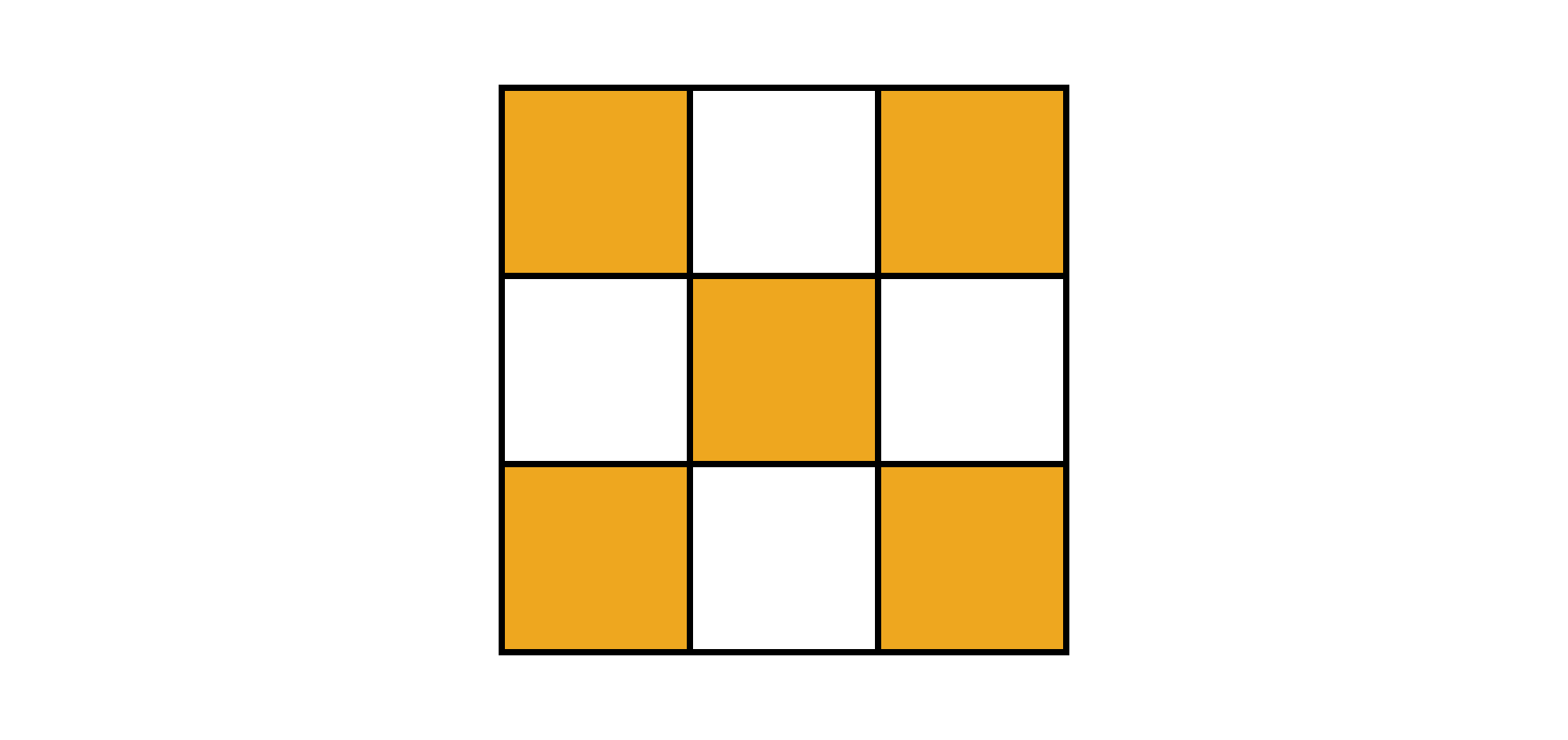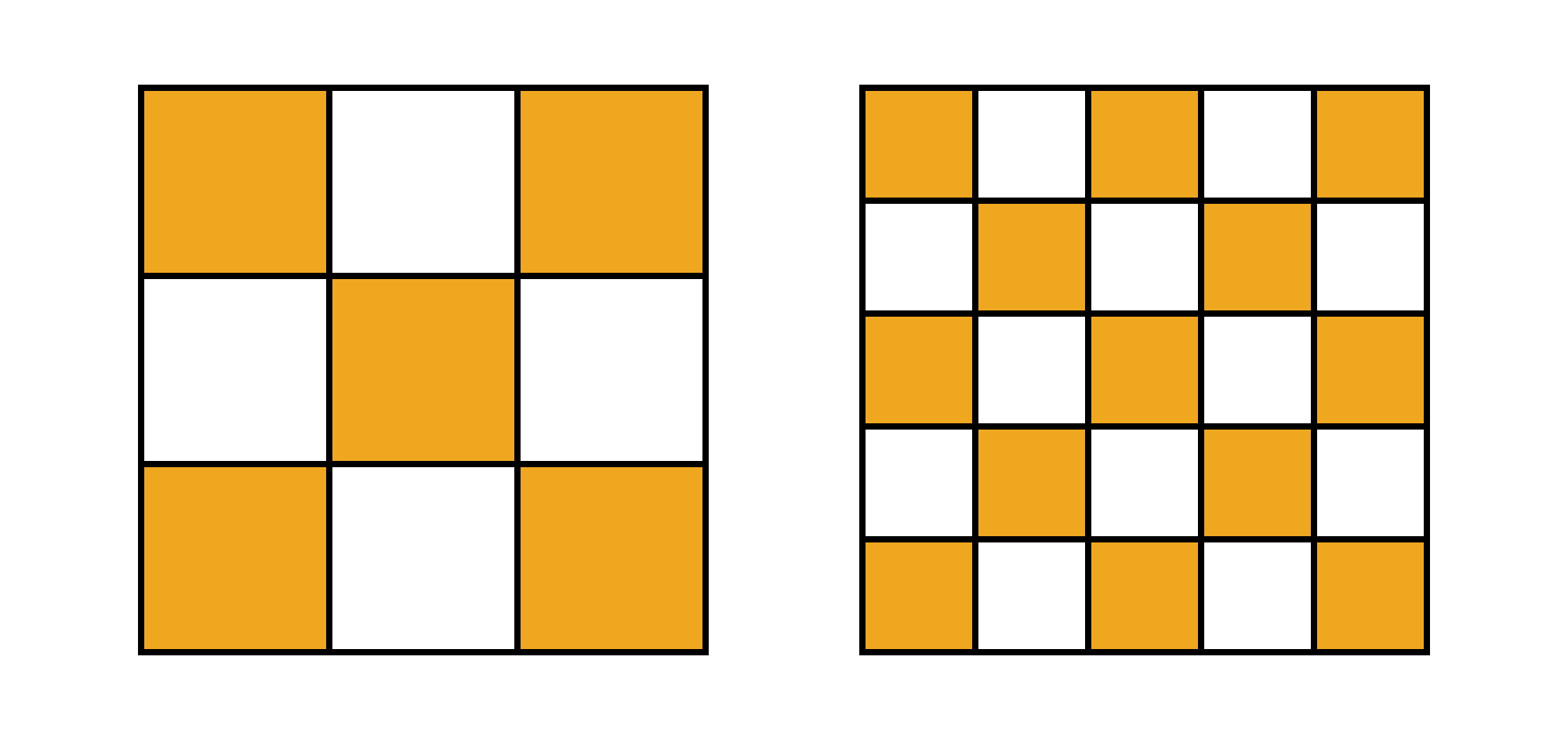### 100 Day Challenge 2020

In the problem below, we have two different squares, which are divided up in ways that look very similar. How can we compare their shaded areas?

Let's consider just the square on the left:This square has been cut into a $3 \times 3$ grid, and five of the small squares are shaded. While we could assign an arbitrary length to the square's side, then divide it by three, and finally calculate the shaded area directly, there's a far simpler way to approach this.

Consider this — there are nine identical squares, and five of them are shaded. Therefore, exactly $\frac{5}{9}$ of the square is shaded.

Can you use this approach to help you compare the two squares below?

# Today's Challenge

Below are two squares of the same size. They've each been divided into many smaller squares:Which of the two large squares has more of its area shaded?